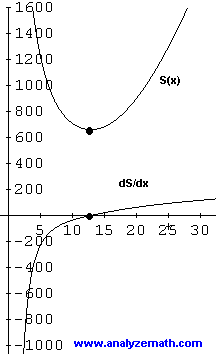# Use First Derivative to Minimize Area of Pyramid Optimization Problem

The first derivative is used to minimize the surface area of a pyramid with a square base.

## Problem

Below is shown a pyramid with square base, side length x, and height h. Find the value of x so that the volume of the pyramid is 1000 cm 3 and its surface area is minimum.Solution to Problem 1:

• This problem has been solved graphically. Here we solve it more rigorously using the first derivative.
• We first use the formula of the volume of a pyramid to write the equation:
(1 / 3) h x 2 = 1000
• We now use the the formula of the surface area of a pyramid to write a formula for the surface area S of the given pyramid. In this problem we have a square pyramid, hence:
S = x * √ [ h 2 + (x/2) 2 ] + x * √ [ h 2 + (x/2) 2 ] + x * x
= 2 x √ [ h 2 + (x/2) 2 ] + x 2
• Solve the equation (1 / 3) h x 2 = 1000 for h to obtain:
h = 3000 / x 2
• Substitute h in the surface area formula by 3000 / x 2 to obtain a formula for S in terms of x (x positive) only and rewrite it as follows:
S = √ [ (36 10 6 + x 6) ] / x + x 2
• Let constant k = 36 10 6 and differentiate S with respect to x.
dS / dx = [ 3 x 6 (k + x 6) -1/2 - (k + x 6) 1/2 ] / x 2 + 2 x
• Multiply numerator and denominator by (k + x 6) 1/2 and simplify.
dS / dx = [ 2 x 6 - k ] / [ x 2 (k + x 6) 1/2 ] + 2x
• A graph of dS / dx is shown below. For x > 0, dS / dx has a zero and is negative to the left of that zero and positive to the right of the zero. This means that S has a minimum value that may be located by setting dS / dx = 0 and solve for x.[ 2 x 6 - k ] / [ x 2 (k + x 6) 1/2 ] + 2 x = 0
• Rewrite as.
[ 2 x 6 - k ] = - 2 x 3 (k + x 6) 1/2
• Let u = x 3 and u 2 = x 6 and rewrite the above as follows:
[ 2 u 2 - k ] = - 2 u (k + u 2) 1/2
• Square both sides:
4 u 4 + k 2 - 4k u 2 = 4 u 2 (k + u 2)
• Simplify to obtain:
k 2 - 4k u 2 = 4 k u 2 k
• Solve for u: (u positive since x positive)
u = √ [ k / 8 ]
• Finally solve for x to obtain:
x = [ √ [ k / 8 ] ] 1/3
• Substitute k by its value and calculate x:
x = 12.8 cm (rounded to 1 decimal place).
• Below are shown the graphs of the surface area and its derivative.More references on calculus problems